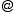LNCS 1879  ESA 2000 Resource Constrained Shortest Paths Kurt Mehlhorn and Mark Ziegelmann mehlhorn@mpi-sb.mpg.de mark.ziegelmann@gmx.de Abstract: The resource constrained shortest path problem (CSP) asks for the computation of a least cost path obeying a set of resource constraints. The problem is NP-complete. We give theoretical and experimental results for CSP. In the theoretical part we present the hull approach, a combinatorial algorithm for solving a linear programming relaxation and prove that it runs in polynomial time in the case of one resource. In the experimental part we compare the hull approach we compare the approach to previous methods for solving the LP relaxation and give an exact algorithm based on the hull approach. We also compare our exact algorithm to previous exact algorithms and approximation algorithms for the problem.
 Impressum & Webmaster last change: 09.02.2011 mark.ziegelmanngmx.de